# Genetic Load of an Evolutionary Change

## Abstract

ACCORDING to Fisher's “Fundamental Theorem of Natural Selection1”, the rate of change of the mean fitness of a population equals the genetic variance in fitness. If w is the fitness and VGw the genetic variance in fitness, then by Fisher's theorem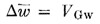Falconer2 showed that if fitness varies in a way that is partially determined by variations in a character, x, then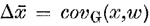where covG(x,w) is the genetic covariance of x and w. This equation Falconer calls the “Extension to Fisher's Fundamental Theorem”. It will be true only if the regression of fitness and the character is linear. That is, we must have a relationship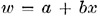where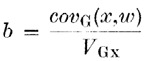It then follows that the genetic load of any change Δ in the mean of the character can be calculated. We have by definition of the genetic load3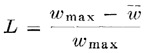where wmax is the fitness of the most fit genotype. Now we have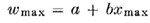and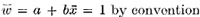It then follows directly that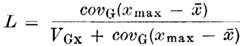If the change per generation in the mean of the character is known, then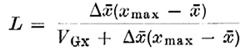The genetic load is an important quantity to know because it measures the intensity of natural selection. If fitness is the probability of survival, then the genetic load is the proportion of the deaths which arise from variations in fitness. Thus it L is large, a population may be in dangerof extinction. For example, suppose a continuous series of fossils shows a change to a new form. During a period of change, we can find Δ if we know the number of generations that has passed. xmax will be the value of the character when the new form has finally become stable. will be the moan at some point in time in the evolutionary process. Thus we can find L at that time. Usually, evolutionary changes occur as populations adapt to new environments. In a new environment xmax will have a new value. The shift of away from xmax produces the genetic load which may be high if VGx is small. The formula for L shows why a large genetic variance helps a population to adapt to an environmental change: if VGx is low the genetic load may be high and the population may become extinct before it can adapt itself. It would therefore be interesting to compare the genetic loads in fossil populations which died out and in those that increased in numbers.

## References

1. 1

Fisher, R. A., Genetical Theory of Natural Selection (Clarendon Press, Oxford, 1930).

2. 2

Falconer, D. S., in Meade, J. E., and Parkes, A. S., Genetic and Environmental Factors in Human Ability (Oliver and Boyd, Edinburgh and London, 1966).

3. 3

Crow, J. F., and Kimura, M., Proc. Eleventh Int. Cong. Genet., 495 (1964).

Authors

## Rights and permissions

Reprints and Permissions

O'DONALD, P. Genetic Load of an Evolutionary Change. Nature 216, 1348 (1967). https://doi.org/10.1038/2161348a0

• Issue Date:

• ### BIRTH CONTROL AND HUMAN EVOLUTION

• R. Cruz-Coke

The Lancet (1968)

• ### SUCROSE INTAKE AND CORONARY HEART-DISEASE

• Denys Jennings

The Lancet (1968)